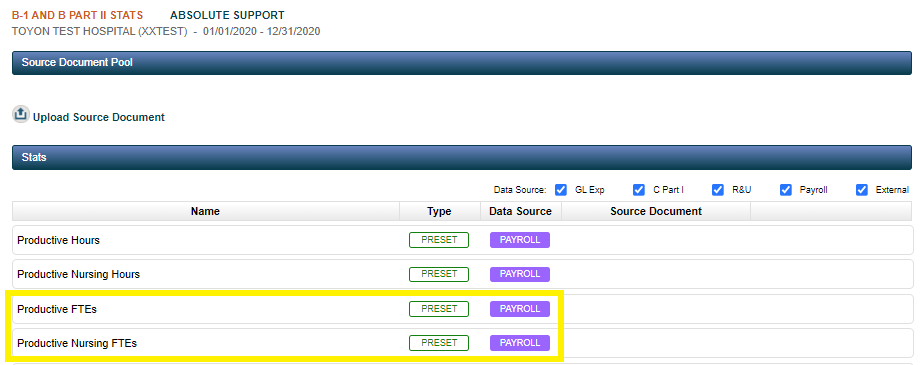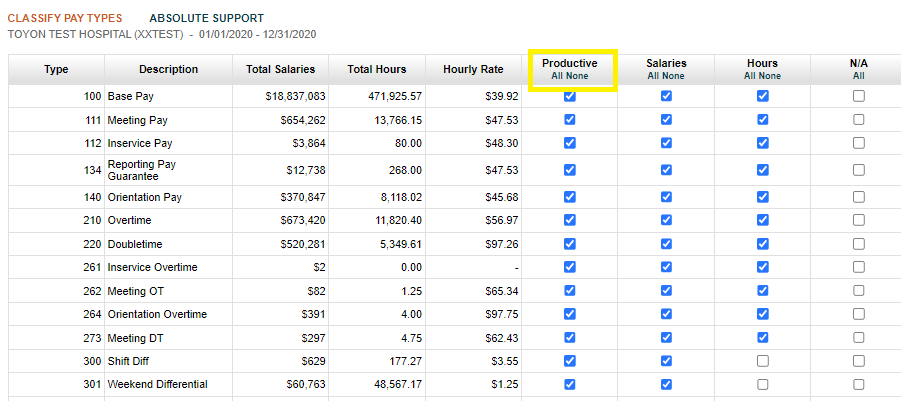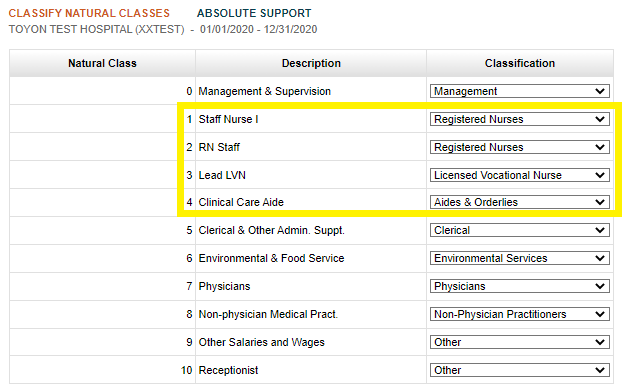# How are the FTEs calculated for B-1 statistics?

As outlined in the B-1 Statistics article, Absolute has been built to include "Preset" statistics which pull data from imported data sources (General Ledger, R&U, and Payroll) in order to create fully automated statistics. This article will explain how Absolute utilizes the Payroll in order to calculate the Productive FTE's as well Productive Nursing FTE's.## Productive FTE's

Absolute calculates the Productive FTEs by looking at the uploaded payroll data (in the Classify Pay Types section) for those pay codes that are marked as Productive.Absolute will also take into account any A-6 reclasses that affect salary, and apply those reclasses to the hours and FTE calculation statistic, to calculate the net hours/FTEs to report for B-1 statistics. To come up with the FTE's, Absolute will take the hours marked as Productive and then divide this number by 2,080.

Finally, Absolute will then multiply the net FTEs x 100 for input into HFS, as HFS won't allow decimals. For example, if a line has 0.4 FTE's, we wouldn't be able to allocate any expense there as it would round to 0.

By design, Absolute multiplies the FTE's by 100 to be as accurate as possible when allocating expense. Because B-1 allocates expense on a percent-to-total basis, multiplying every line by 100 won't have an effect on the ratio used to allocate total expense. It will only make the allocation more accurate.

## Productive Nursing FTE's

Absolute calculates the Productive Nursing FTE's using the same logic outlined above, but will now incorporate the Job Code Classifications that were mapped in the Classify Job Codes section. Further, Absolute will only include Productive hours that belong to Job Codes classified as Registered Nurses, Licensed Vocational Nurses, and Aides and Orderlies in the FTE calculation.#### More Resources

For more information B-1 Statistics, see the following article: B-1 and B Part II Statistics

For B-1 Statistic Troubleshooting, see the following articles: Why can't I mark Worksheet B-1 Complete? & Why aren't my B-1 Preset Statistics for Productive FTEs/Hours populating?